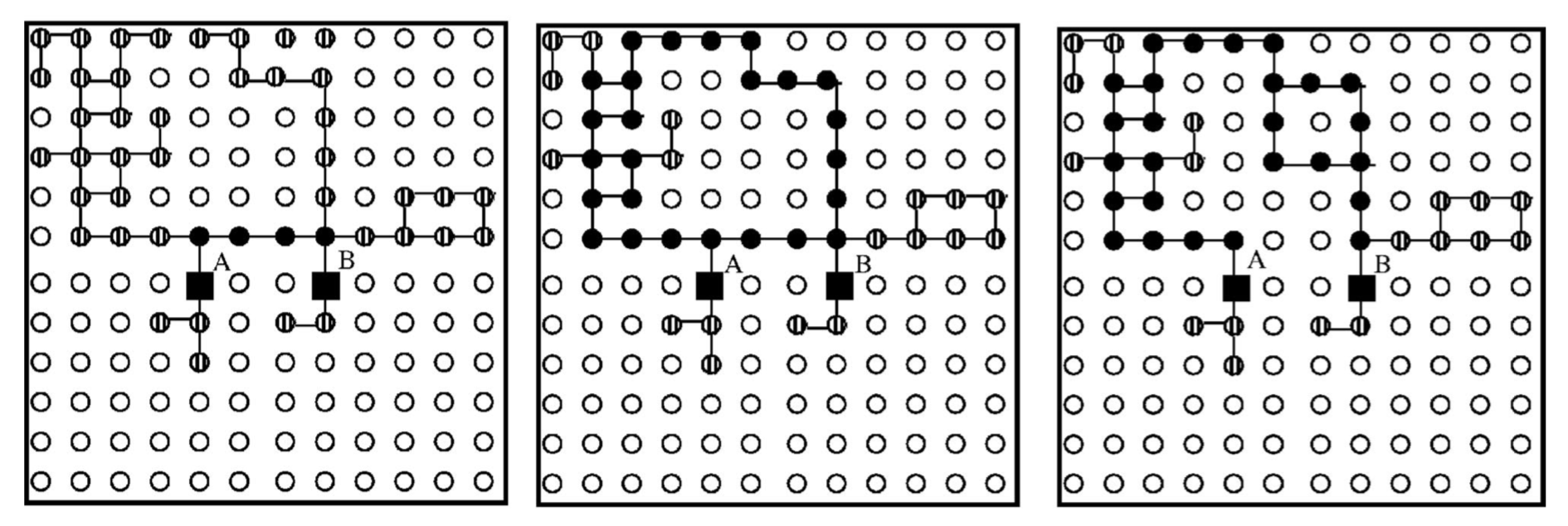# Figuring out a consistent definition for the percolation backbone

In the context of percolation, e.g., bond/site percolation, random graph connectivity in 2-3 dimensions, etc., once the percolation threshold is reached, that is the system is spanned by an infinite cluster (or in the finite case a cluster connecting two boundaries of the system), the behaviour of backbone of this cluster may be of particular interest. In general, skimming through various related literature one comes across slightly differing definitions for the backbone that tend to coincide. To name a few formulations for the backbone:

a) Suppose we have two vertices $$i$$ and $$j,$$ if we apply a potential difference between them, then the set of current carrying bonds (bonds of the percolating cluster having non-zero flow of current) between $$i$$ and $$j$$ is called the backbone, and the removed ones are dangling ends. In other words, the backbone is a subset of the percolation cluster formed by removing all dangling ends, branches, etc. that are not necessary for maintaining the connectivity of the structure.

• The definition in a) given in terms of current flow between two nodes (so defined closely related to flow problems), does not seem to exclude the possibility of having loops/cycles in the backbone? But given Kirchhoff's second law, namely that the sum of all potential differences around a complete loop is zero, such loops are trivially dismissed (?) in the definition of a), or possibly their inclusion would bear no difference on the properties of the backbone of the percolating cluster.

b) However, leaning more on a mathematical definition, the backbone is also sometimes defined as a cluster connecting $$i$$ and $$j$$ such that it can be explored by a self-avoiding walk. On the other hand, sometimes it is given a more practical definition related to shortest path between $$i$$ and $$j,$$ namely, a backbone can be found by choosing a vertex randomly on the cluster (connecting $$i$$ and $$j$$) and retaining only the vertices and bonds that belong to the shortest path between them. Finally, more descriptively, the backbone is also described as the part of the percolating path that is free of cycles and dangling ends.

• Compared to a), the types of definitions in b) seem to suggest more clearly that loops are not taken as part of the backbone (as e.g. it would undermine the self-avoiding walk). Similarly, when defined in relation to the shortest path, the loops would become irrelevant.

Question:

• Given the definitions in a) and b), can a consistent definition be drawn for what is meant by the backbone of a percolating cluster? Are the existence of these somewhat differing definitions a case of rigour versus practicality?
• On the computationally practical side, what would be a reasonable working definition for the backbone?

I admit I may have mis-interpreted the above definitions (e.g. the loop aspect when considering current flow between $$i$$ and $$j$$), or the self-avoiding walk description, which to my understanding, would dismiss dangling ends and loops from the backbone.